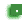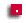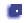# While Loop programs

Read the class notes on while loops. Read the section on break; continue;
Copy the program at the bottom of the while loop class note page and run it.

## There are three (plus one) programs to do:

###While50.java (everybody do this)

Write a while loop that will print out the numbers 1-50 (i.e. it loops 50 times printing out a number)

This is just marked for completion

###GuessNumber.java (everybody do this)

Write a guess a number game.
a) make a random number between 1 and 100 using this code: `int secret = (int) (Math.random() * 100) +1;`
b) when the user enteres a guess, say if it is too high, too low or just right.
c) print out the number of guesses once the user wins.

This will be marked more carefully for formatting, variable names, etc.

###While99.java (everybody do this)

Start learning how to use `break;` and `continue`

• Your program will get numbers (int) from the user. Do this in a while loop so that you keep getting more numbers.
• If the number is a multiple of 3 or 5, do nothing (use the "continue" command for this)
• If the number is -99 then exit the loop
• All other numbers should be added to the total (yes, you're making a total of all of the numbers)
• After you exit the while loop print out the total.

Here's the outline of how to do it (but there is one problem you'll have to fix to get it to work)

• make a scanner
• create a total variable
• start while loop: `while (true) {`
• get number from user (print something first)
• if number is a multiple of 3 or 5 continue. Use `if (num%3 == 0) continue;`
• if number is -99 then break;
• add number to total and print the total
• end the loop `}`
• print out total

Example:

```n	total
---------------
2	2
4	6
20	6	(multiples of 5 are ignored)
11	17
6	17	(multiples of 3 are ignored)
-99	17	(exit the loop)

Total = 17```

###PlusMinus.java (enrichment)

Write a program that gets numbers (positive integers) from the user. Alternate between adding and subtracting each successive number. Print out the total each time. Quit when the total equals 100.

###While_q.java (Try and do this, but not marked)

• Write a program with a while loop that gets a string from the user
• Make the condition in the while loop a boolean variable.
• Check the first letter in the word that the user types in:
```String s = keyboard.next(); char letter = s.charAt(0); ```
• If the letter is a 'q' then exit the loop
• exit the loop by changing the boolean
• then try and exit the loop by using "break"
• Now rewrite the while loop to be a do-while loop